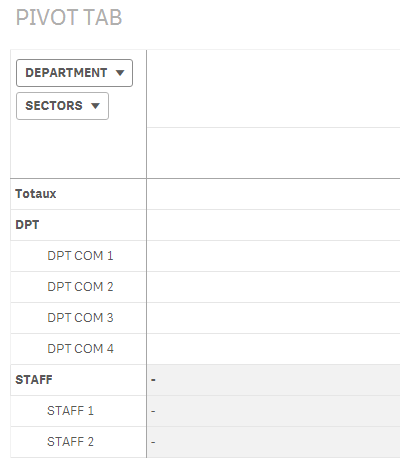# App Development

Announcements
April 22, 2PM EST: Learn about GeoOperations in Qlik Sense SaaS READ MORE
cancel
Showing results for
Did you mean:Creator II

## If(dimensionality () = 0 & 1 ?

Hi everybody

i have a PIVOT TAB like thisI created Aggr expressions with ratios and i use IF( dimensionality () for TOTAL and SUBTOTAL calculations like this

If(Dimensionality() = 0,

Sum(Aggr( .....

If(Dimensionality() = 1,

Sum(Aggr(....,

it's working perfectly, but i think it's a too complicated syntax

Is there a syntax that alows me to write only one time the expression (as the fonction "OR" in excel) ?

like this :

If(Dimensionality() = 0, OR 1,

Sum ( Aggr( ...

Regards

Philippe

1 Solution

Accepted SolutionsMVP

>>Is there a syntax that alows me to write only one time the expression (as the fonction "OR" in excel) ?

If(Dimensionality() = 0 Or Dimensionality() = 1, .....)

Logic will get you from a to b. Imagination will take you everywhere. - A Einstein
3 RepliesMVP

If the aggr() includes all the chart dimensions, then you would not need a separate logic for the subtotals and totals, so you would not need the Dimensionality() split. Unless, of course, you need different logic, like and Average at the totalling levels and a sum at the detail levels,

Logic will get you from a to b. Imagination will take you everywhere. - A EinsteinMVP

>>Is there a syntax that alows me to write only one time the expression (as the fonction "OR" in excel) ?

If(Dimensionality() = 0 Or Dimensionality() = 1, .....)

Logic will get you from a to b. Imagination will take you everywhere. - A EinsteinCreator II
Author

Hi Jonathan

in my case

If(Dimensionality() = 0 Or Dimensionality() = 1

is  all right !

regards

Philippe